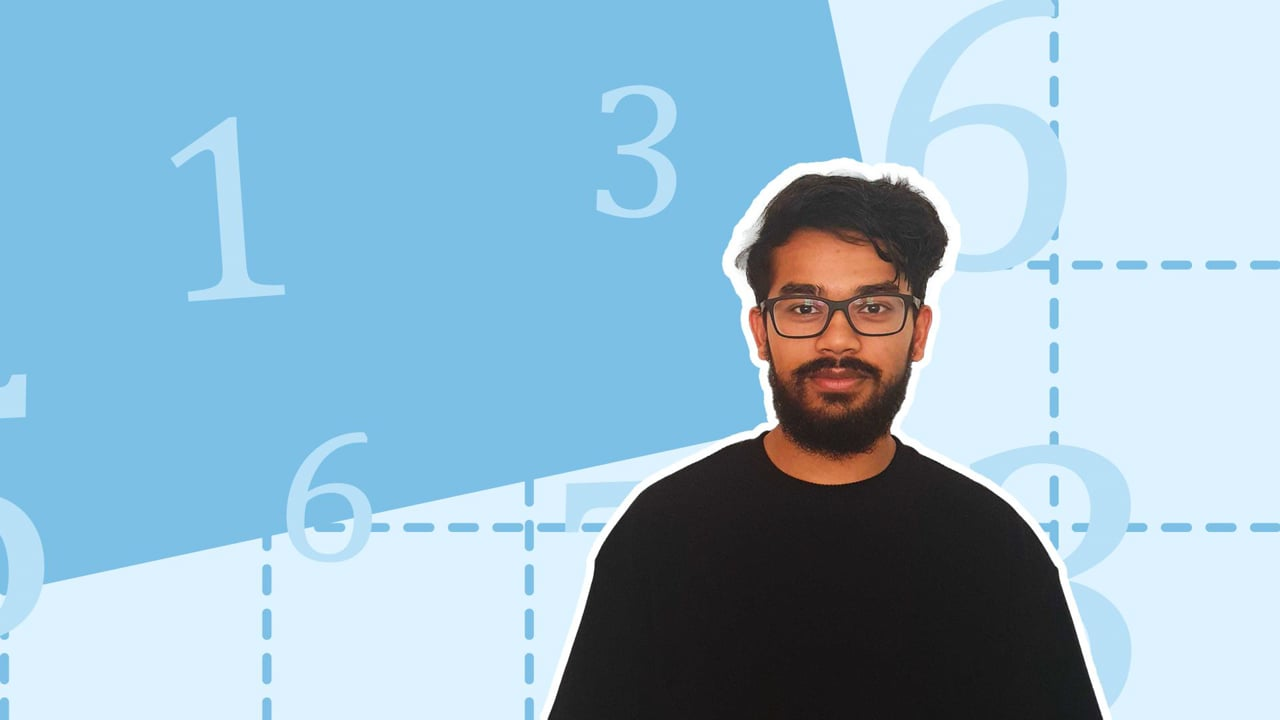Chapter overviewMaths

Number and place value

Multiplication and division

Fractions

Measurement

Geometry - properties of shapes

Geometry - position and direction

Statistics

Maths

# Prime numbers, prime factors and composite numbers0%

Summary

# Prime numbers, prime factors and composite numbers

## In a nutshell

Prime and composite numbers are special categories of numbers based on how many factors they have. There are $25$ prime numbers and $74$ composite numbers between $1$ and $100$.

## Prime numbers and composite numbers

Prime numbers only have two factors, $1$ and itself. Composite numbers have more than two factors.

##### Example 1

Identify whether the following numbers are prime or composite: $2,10,13,25$.

 Number Factors Prime or Composite ​$2$​​ ​$1,2$​​ ​$Prime$​​ ​$10$​​ ​$1,2,5,10$​​ ​$Composite$​​ ​$13$​​ ​$1,13$​​ ​$Prime$​​ ​$25$​​ ​$1,5,25$​​ ​$Composite$​​

​​

Note: $1$ is neither a prime or composite number because it only has one factor, $1$

## Prime factors

Factors of a number which are prime are prime factors. They can be found using a factor tree to breakdown a number into its prime factors which can be multiplied together to give the original number. This can be done by following the procedure below:

#### PROCEDURE

 ​$1$​​ Write down the given number at the top. ​$2$​​ Find any two numbers which multiply to give the top number. ​$3$​​ Write down these two factors beneath the original number at the end of a left and right "branch". ​$4$​​ Repeat this process for all the numbers in the tree until each "branch" ends in a prime factor. ​$5$​​ ​Circle each prime factor, and write them all multiplied by each other.

##### Example 2

What is $32$ as a product of its prime factors?

Write $32$​ on the top, and find two numbers that multiply to give $32$:

$32=2\times16$

​​

Write $2$ and $16$ beneath $32$$2$​ is a prime number so circle it.

{\begin{aligned} &\space \space \,\,\,\,32\\\ &\,\,\swarrow\searrow& \\ &\textcircled{2} \quad16 \end{aligned}}​​

As $16$ is not a prime number, find two numbers that multiply to give $16$ such as: $2 \times 8$​. Add this to the factor tree and circle $2$ since it is prime. ​

\quad{\begin{aligned} &\space \space \,\,\,\,32\\\ &\,\,\swarrow\searrow& \\ &\textcircled{2} \quad16 \\ &\quad\,\,\swarrow\searrow\\ &\space\space\space\,\,\textcircled2 \,\,\quad8 \end{aligned}}

​​

As $8$ is not a prime number, find two numbers that multiply to give $8$ such as: $2 \times 4$​. Add this to the factor tree and circle $2$ since it is prime.

\quad\quad{\begin{aligned} &\space \space \,\,\,\,32\\\ &\,\,\swarrow\searrow& \\ &\textcircled{2} \quad16 \\ &\quad\,\,\swarrow\searrow\\ &\space\space\space\,\,\textcircled2 \,\,\quad8 \\ &\quad\quad\,\,\,\swarrow\searrow \\ &\quad\space\space\,\,\textcircled2 \quad\space\,\,\, 4 \end{aligned}}​​

​As $4$​ is not a prime number, find two numbers that multiply to give $4$​ such as $2 \times 2$​. Add this to the factor tree and circle both $2$s since they are prime.

​​

\quad\quad\quad\space{\begin{aligned} &\space \space \,\,\,\,32\\\ &\,\,\swarrow\searrow& \\ &\textcircled{2} \quad16 \\ &\quad\,\,\swarrow\searrow\\ &\space\space\space\,\,\textcircled2 \,\,\quad8 \\ &\quad\quad\,\,\,\swarrow\searrow \\ &\quad\space\space\,\,\textcircled2 \quad\space\,\,\, 4 \\ &\quad\quad\quad\space\,\,\swarrow\searrow \\ &\quad\quad\quad\textcircled2 \quad\space \,\,\textcircled2\end{aligned}}​​

Only prime factors remain so the tree is complete.

​​​

Write $32$ as a product of its prime factors.​

Therefore, $\underline{2 \times 2\times 2\times 2\times 2=32.}$

Note: $1$ is not a prime number so it does not appear in the factor tree.

FAQs

• Question: What are prime numbers?

Answer: Prime numbers only have two factors, 1 and itself.

• Question: What are composite numbers?

Answer: Composite numbers have more than two factors.

• Question: Is 1 a prime number?

Answer: 1 is not a prime number because it only has 1 factor which is 1.

Theory

Exercises